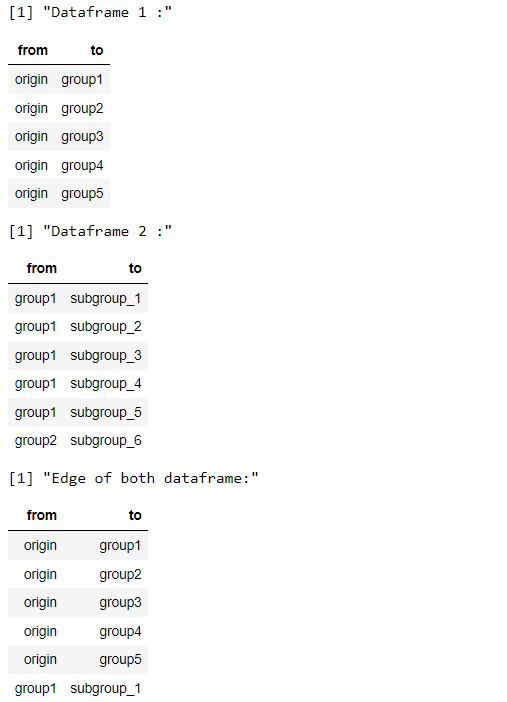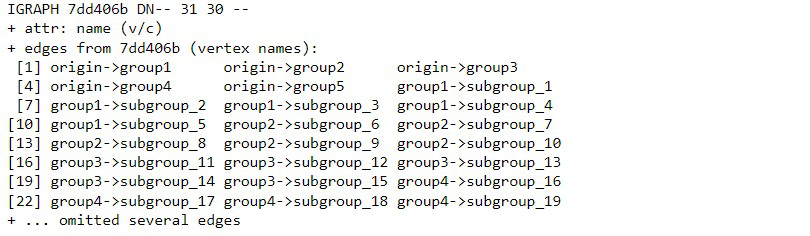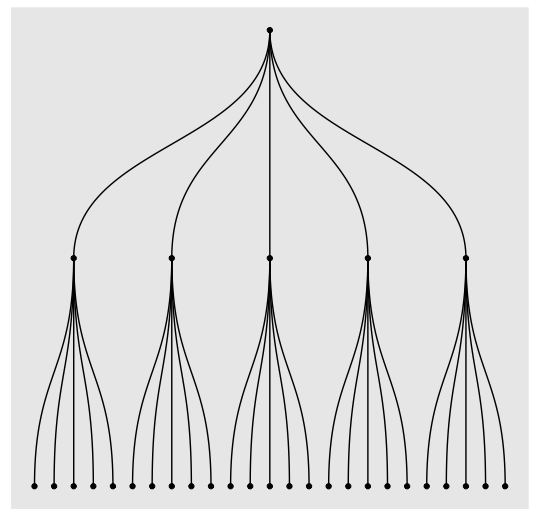# Introduction to tree diagram with R and ggraph

• Last Updated : 20 Feb, 2022

In this article, we are going to see how to visualize the tree diagram with R Programming using ggraph library. ggraph library has a bunch of methods to help in visualization in R Programming Language.

## Visualizing the Dendrogram

A dendrogram is a visual representation of the hierarchical relationship between items. It’s most often produced as a result of hierarchical clustering. A dendrogram’s main purpose is to figure out the best approach to assign objects to clusters.

### Preparing the Hierarchical Data

Here we are going to prepare hierarchical data for demonstration. For this, we will create two dataframe, and then we will create edge data.

## R

 `# libraries for plotting the graph ` `library``(ggraph)  ` `library``(igraph) ` `library``(tidyverse) ` `library``(ggplot2) ` ` `  `# create the edge data for the hierarchy ` `d1 <- ``data.frame``(from = ``"origin"``,  ` `                 ``to = ``paste``(``"group"``, ` `                            ``seq``(1,5), ` `                            ``sep = ``""``)) ` `print``(``"Dataframe 1 :"``) ` `head``(d1) ` ` `  `# preparing the subgroup data ` `d2 <- ``data.frame``(from = ``rep``(d1\$to, each = 5),  ` `                 ``to = ``paste``(``"subgroup"``,  ` `                            ``seq``(1,25), ` `                            ``sep=``"_"``)) ` ` `  `print``(``"Dataframe 2 :"``) ` `head``(d2) ` ` `  `# creating the edge data ` `edges <- ``rbind``(d1, d2) ` ` `  `print``(``"Edge of both dataframe:"``) ` `head``(edges)`

Output:### Prepare the Graph Data

graph_from_data_frame() methods creates igraph graphs from one or two data frames and its descriptions have been obtained from the igraph package.

Syntax: graph_from_data_frame(d, directed = TRUE)

Parameters:

• d : Dataframe
• directed: Logical scalar

## R

 `# prepare the graph data ` `mygraph <- ``graph_from_data_frame``( edges ) ` `mygraph`

Output:### Preparing the Dendrogram

Now, we will plot dendrogram using ggraph library. For this, we will use dendrogram layout of ggraph with layout = ‘dendrogram’  and geom_edge_diagonal() is used to the edges and geom_node_point() for data points

Syntax: ggraph(graph, layout)

Parameters:

• graph: graph Object
• layout: Layout type instring, a function, a matrix, or a data.frame

## R

 `# plotting the dendrogram ` `ggraph``(mygraph, layout = ``'dendrogram'``) +  ` `  ``geom_edge_diagonal``() + ``# putting up the edges ` `  ``geom_node_point``() ``# add up the data points`

Output:My Personal Notes arrow_drop_up
Recommended Articles
Page :